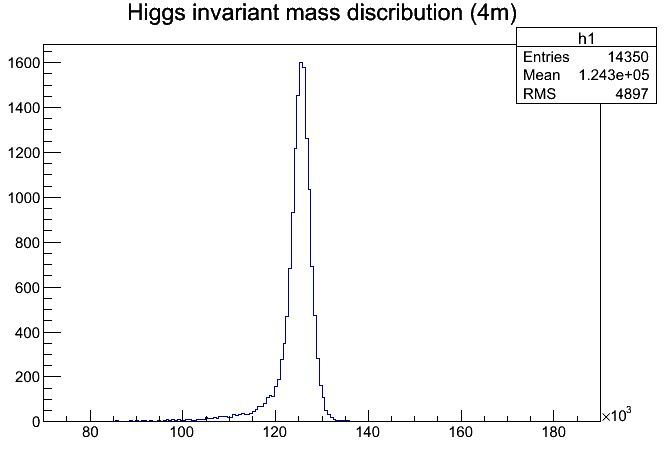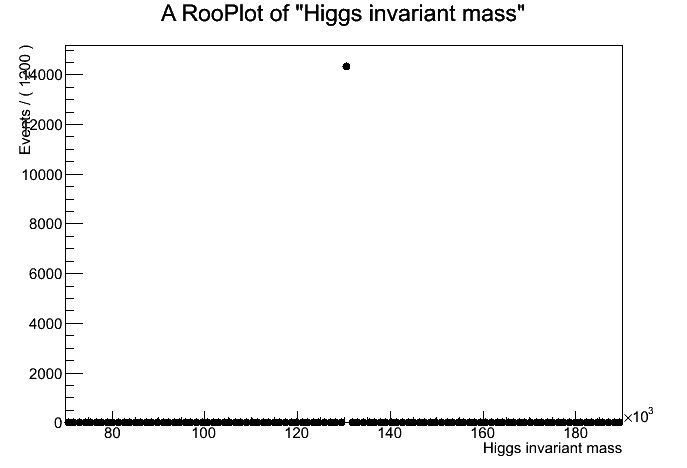# A Problem About Importing Data Within RooFit

Hello, everyone!
I’m using RooFit to fit some histograms, but there is a problem haven’t been solved yet. I followed the RooFit guide to import unbinned data from a tree, but it seems that something went wrong, the figure I got is wrong. Here is my root script:

[code]Higgs_4m_Draw(){
TFile* input = TFile::Open(“datalist01_smearing.root”);
TTree* tree = input->Get(“Higgs_4m”);//there is a tree named “Higgs_4m” in the root file

TH1F* h1 =new TH1F(“h1”,“Higgs invariant mass discribution (4m)”,200,70e+3,190e+3);
tree->Project(“h1”,“Hmass”);
TCanvas *c1 = new TCanvas(“c1”,“new canvas”,1);
h1->Draw();

using namespace RooFit;
RooRealVar Hmass(“Hmass”, “Higgs invariant mass”,70e+3,190e+3);
RooDataSet data(“data”,“Hmass”,tree,Hmass);//“Hmass” is a variable in the “Higgs_4m” tree

TCanvas c2 = new TCanvas(“c2”,“new canvas”,1);
RooPlot
Hframe=Hmass.frame();
data.plotOn(Hframe);
Hframe->Draw();
}[/code]
And here are the two pictures I got, the second one is problematic. I just don’t understand why. Do you have any idea about that ? Thanks a lot!cheers,
John